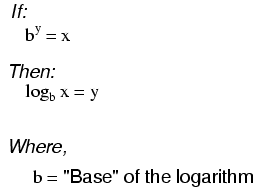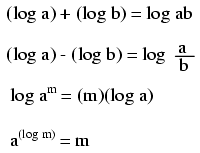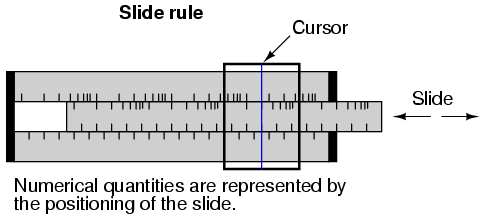# Learning Electronics

Learn to build electronic circuits

# Logarithms

### Definition of a logarithm"log" denotes a common logarithm (base = 10), while "ln" denotes a natural logarithm (base = e).

### Properties of logarithmsThese properties of logarithms come in handy for performing complex multiplication and division operations. They are an example of something called a transform function, whereby one type of mathematical operation is transformed into another type of mathematical operation that is simpler to solve. Using a table of logarithm figures, one can multiply or divide numbers by adding or subtracting their logarithms, respectively. then looking up that logarithm figure in the table and seeing what the final product or quotient is.

Slide rules work on this principle of logarithms by performing multiplication and division through addition and subtraction of distances on the slide.Marks on a slide rule's scales are spaced in a logarithmic fashion, so that a linear positioning of the scale or cursor results in a nonlinear indication as read on the scale(s). Adding or subtracting lengths on these logarithmic scales results in an indication equivalent to the product or quotient, respectively, of those lengths.

Most slide rules were also equipped with special scales for trigonometric functions, powers, roots, and other useful arithmetic functions.